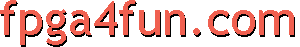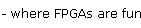## CNC 5 - FPGA motion controller

##### FPGA design

Here's the FPGA motion controller block diagram (three axes shown):The USB-2 data gets buffered in a FIFO and then goes to a demultiplexor. Since the data is "packetized", the demultiplexor is necessary to distribute the acceleration data to each integrator's axis. After the integrators, pulse generators make sure the Step/Dir pulses have the proper timing.

Here's the integrator's heart.

```generate
for(i=0; i<nbaxes; i=i+1)
begin:motion
always @(posedge clk) axes_S[i] <= axes_S[i] + axes_A[i];
always @(posedge clk) axes_P[i] <= axes_P[i] + axes_S[i];
end
endgenerate
```